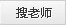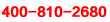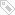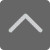爱智康资讯站

课程咨询: 4000-121-121一年级上册数学期末试卷-小学数学一年级上册第九单元测试题

2019-01-09 23:04:37 　来源：网络整理

一年级上册数学期末试卷-小学数学一年级上册第九单元测试题你已经一年级了，你的口算能力有没有进步？下面就是小编为大家带来的一年级上册数学期末试卷-小学数学一年级上册第九单元测试题，希望可以帮助到大家。

我会算

9+5= 16-6= 3+10= 3-2= 8+9=

4+8= 19-10= 3+8= 9+3= 9-6=

7+7=　　 6+5= 14-2=　　7+4=　 9+1=

9+5= 5+8= 15-5= 7+4= 7-5=

0+18= 13-3= 7-4= 19-5= 18+2=

2+9= 7+5= 9+3= 8+5= 4+9= 9+4=

7+5= 15-0= 10-7= 8+4=

9+2= 4+8= 3+8= 4+6= 8+3=

5+7= 15-4= 14-0= 5+7= 9+8=

4+5= 7-2= 12+5= 4+8= 5-5=

6+8= 12-1= 9+4= 13-5= 7+5=

9+6= 5+9= 15-5= 9-7= 9+5=

6-5+8= 9- 9+15= 6-2+3= 9-3+7=

9+4-4= 2+ 5+8= 4+5-3= 10-2+4=

10-9+19= 4+4-2= 15-0-5= 6+4-8=

1+5+2= 3+ 5+2= 15-5-9= 7+4-1=

3+4+1= 9-7+6= 15-5+9= 3+7+4=

小编推荐：

以上就是小编特意为大家整理的一年级上册数学期末试卷-小学数学一年级上册第九单元测试题希望对有需要的同学提供帮助，大家如果在学习中有什么疑问，欢迎拨打爱智康免费电话：!那里有专业的老师为大家解答。

2019年精品学习包：

•小学语|数|英

历年试卷、北京30所校练习题、名著|重点校巡礼|等100讲视频、纸质书籍领取

•初中九大学科

历年真题、期末|期中|志愿填报|重点校巡礼|等100讲视频、纸质书籍领取

•高中九大学科

历年真题、期末|期中|志愿填报|重点校巡礼|等100讲视频、纸质书籍领取

•更新中

学习包内容持续更新中......标签：• 全国课程在线咨询
• 咨询热线：4000-121-121
• 扫描注册有礼

• 让学习更有效